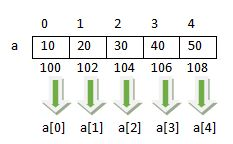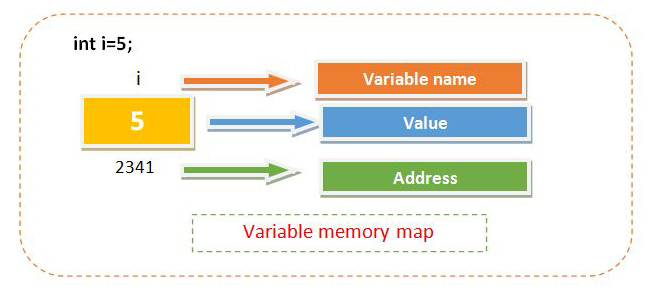## Posts

Showing posts from March, 2019

### Arrays in C LanguageSuppose you want to store the 10 numbers. The one choice is to create a 10 different variable but it is difficult when number of variable are increases. So the next solution that is more feasible is array. An array is the collection of various similar type elements stored in contiguous memory locations. All the array elements share the common name. To uniquely identify each element an index or subscript is associated with the element. so the array is also known as subscript variable. In the given figure arrangement of array elements are shown. The address of the first element is 100. If the given array is of type integer and integer occupies 2 bytes then the next element in the array has address 102,104 etc as given in the figure. How to access array elements using subscript operator it is also shown in the given figure.Array index always start with 0. To access array element subscript operator is used.[] is called subscript operator.a read a of 0 or 0th element of aCreating/Declar…

### RecursionA function is generally call another function to accomplish the task but sometimes a function can call itself to complete the task. This type of function is known as a recursive function and this feature is known as recursion. Let's take a short example of the recursive function.main(){ printf("Hello"); main(); }In the above program snippet main() function calls itself so main() is known as recursive function but the output of the above program snippet is "hello" prints indefinitely. The following program snippet improve the above logic and try to make it finite. main() { int i=1; if(i<=10) { printf("Hello"); i++; main(); } } If you thought that above logic print "Hello" 10 times then you are wrong because when program start i becomes one and of course the condition gets true and "Hello" gets printed then i becomes 2 by increment and then the main() function is again called. As the main()…

### Pointers in C LanguagePointer is called the soul of c. The importance of pointer can be understood by the fact that most of the device driver or embedded system program are created in c. Pointer makes the c most usable and flexible programming. Due to the availability of the pointer, it is possible to write a program such as a device driver. These programs are also known as the device driver. To understand the pointer we have to first understand the memory organization of any variable. The following figure shows the fact. Whenever a variable stored in memory it occupies some memory space and every memory space has a unique number associated with it known as an address. Every byte in member has a different address. If we create an integer variable and suppose it occupy 2 bytes in memory, then two addresses are associated with it but its first address is enough to access it. so the address of next bytes is less useful. To access the address of a variable & (Address of) operator is used. To understand the…

### Functions in C Language

Function is a group of statements which perfoms an specific task.We have used many functions such as printf(),scanf() and many more while developing a program. The purpose of a function can be understood by the example of printf(). Whenever you want to print anything on the output screen you use the printf() function. Actually to send the output on the screen is a difficult task and a program of multiple statements is need to write but fortunately, this is already done by Dennis Ritchie. So you can print anything by using a single line.Types of functionIn c various function are already created such as printf(),scanf(),sqrt(),pow() etc.These are known as predefined function or library function.C also provide the facility to create your own function. The functions created by the programmer are known as user defined function.Every function is followed by a pair of parenthesis(). While working with a function, some input needs to be supplied to the function. The input is given by writing …